Anzeige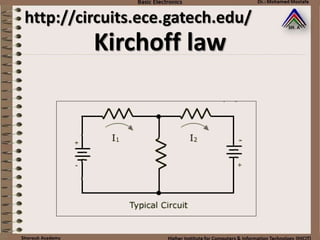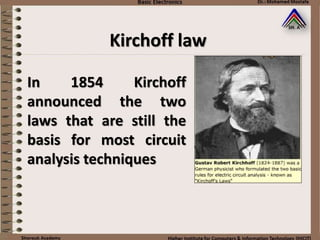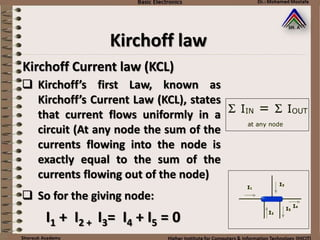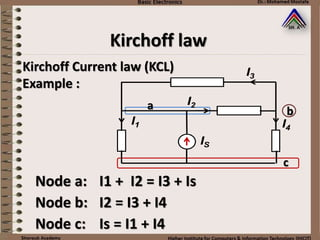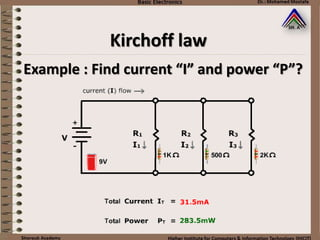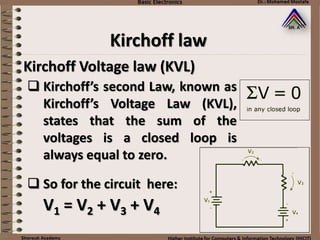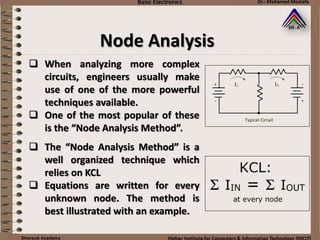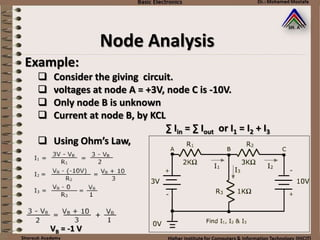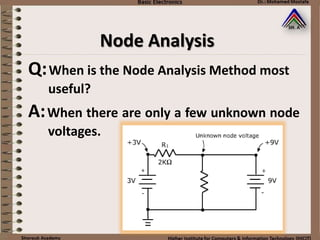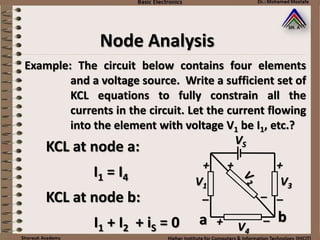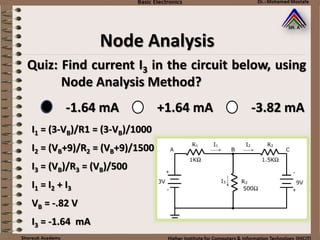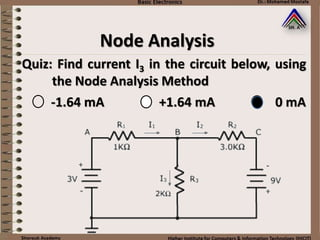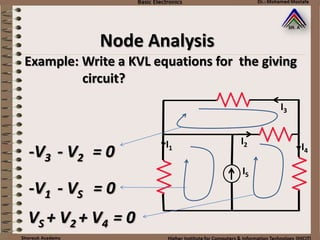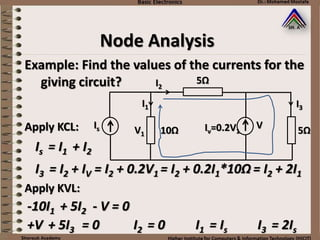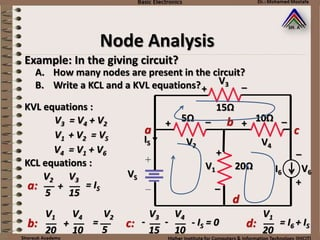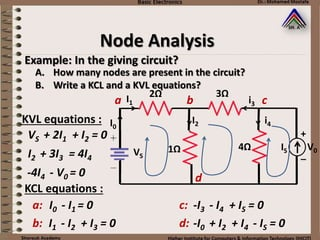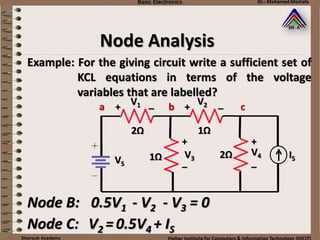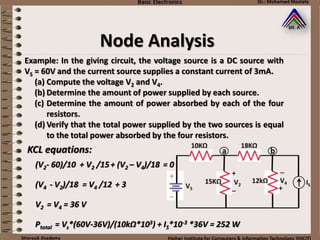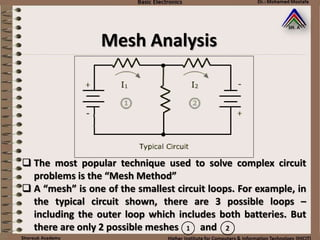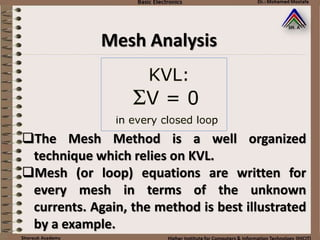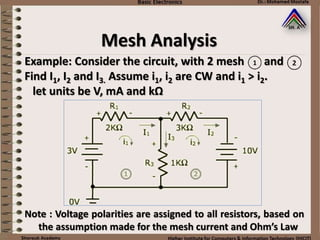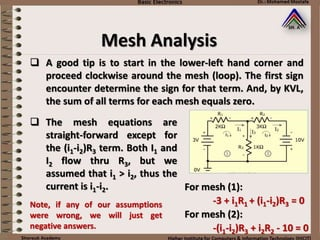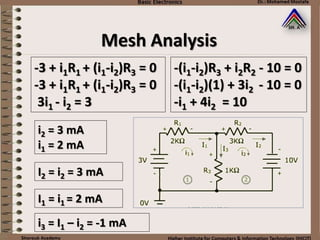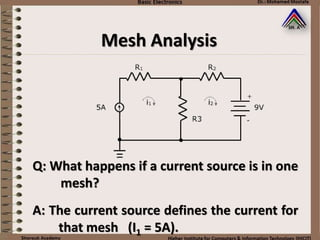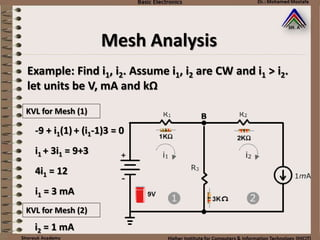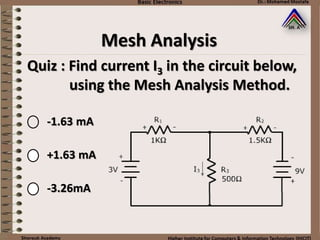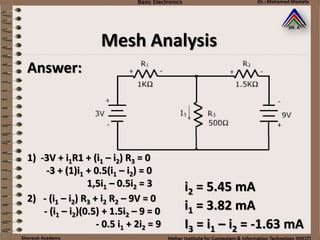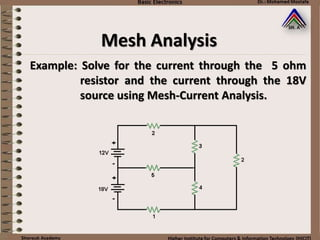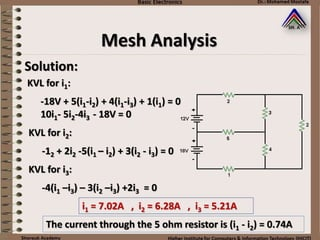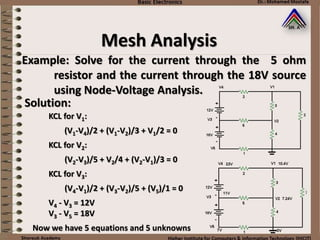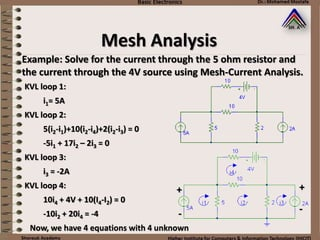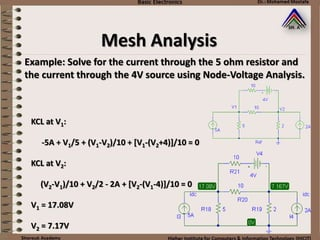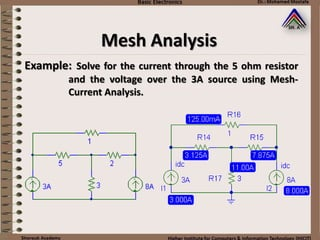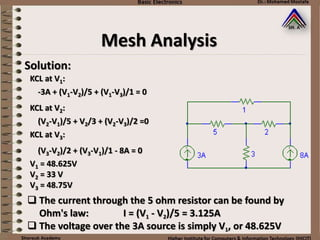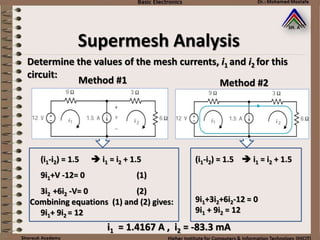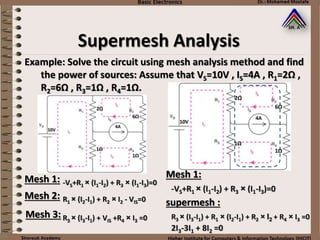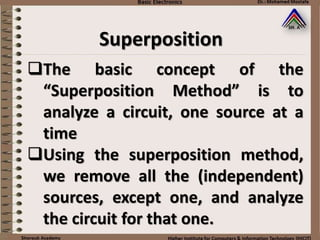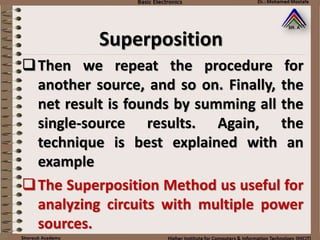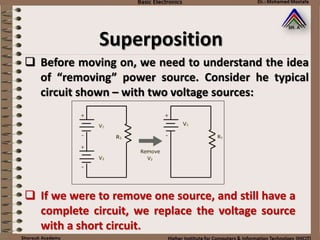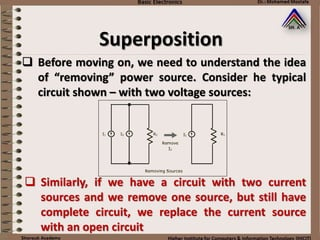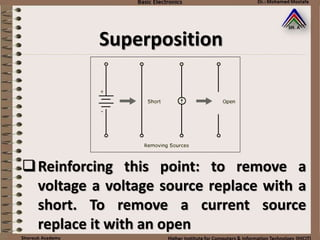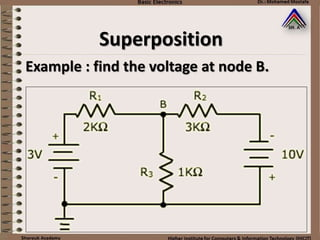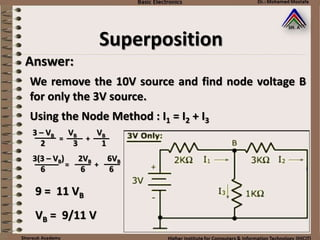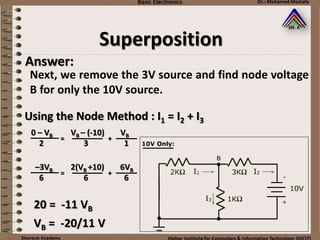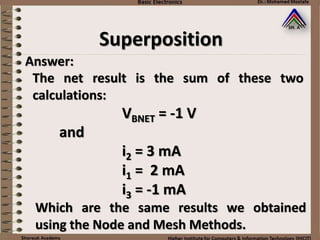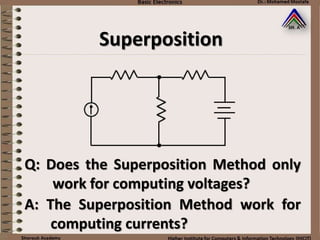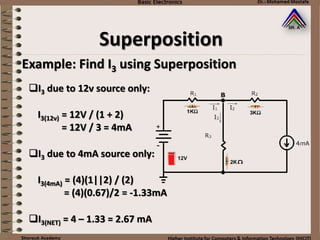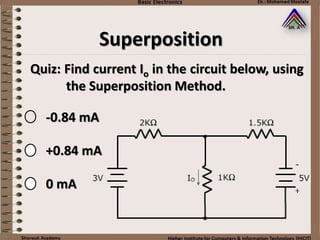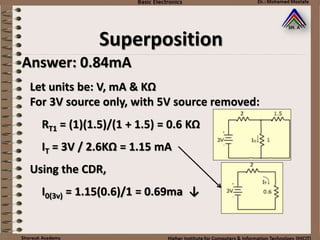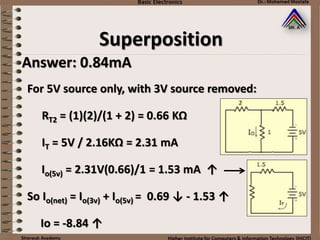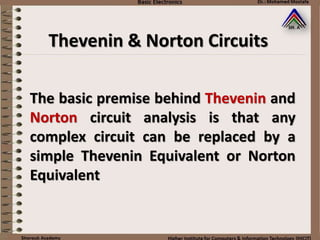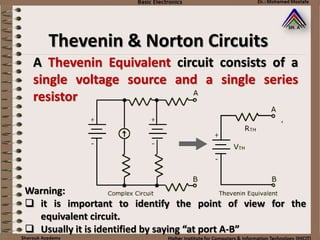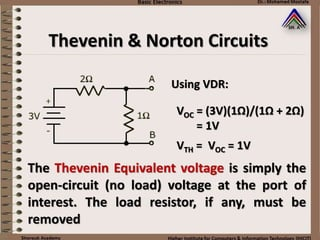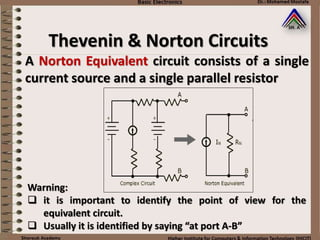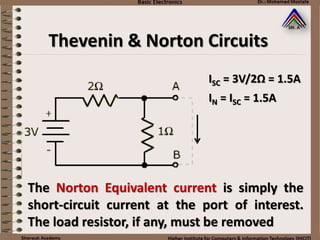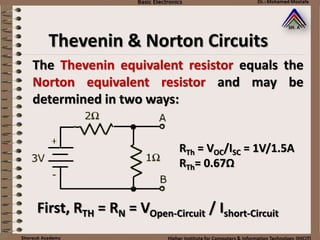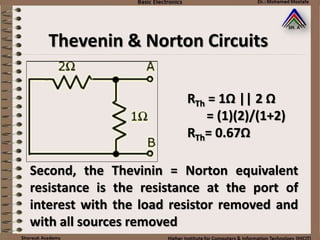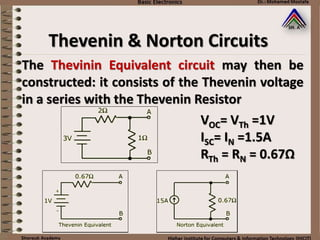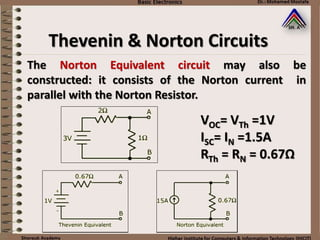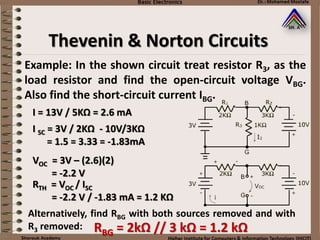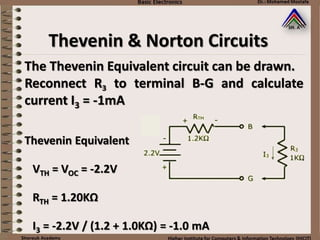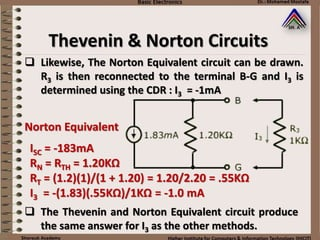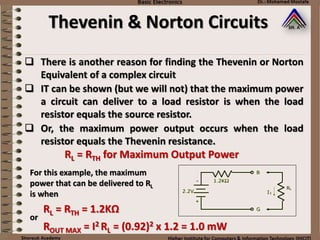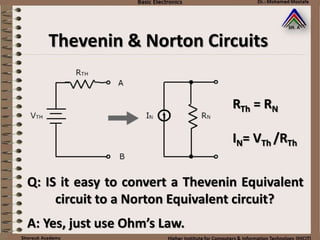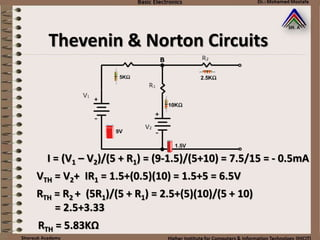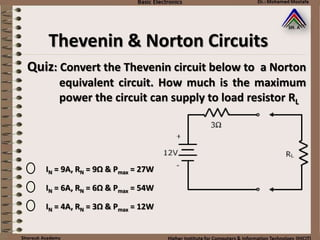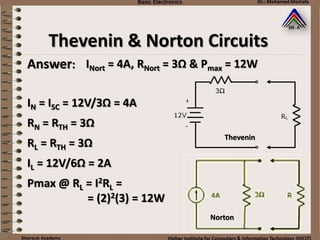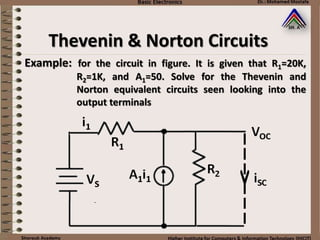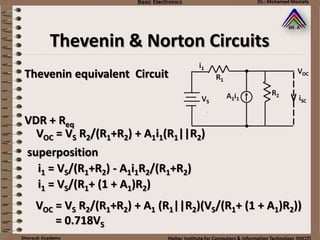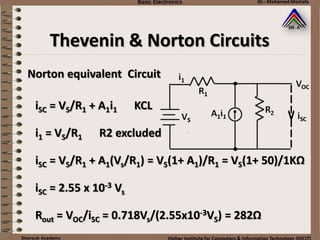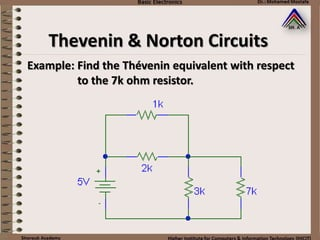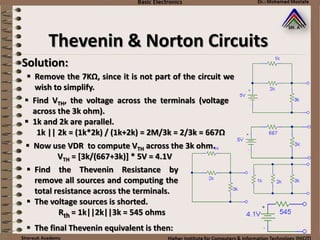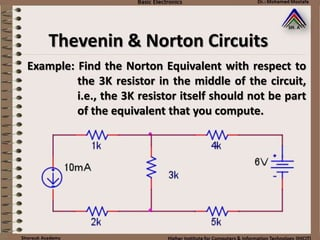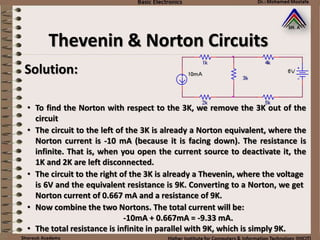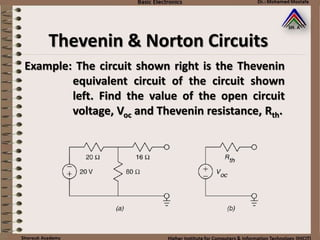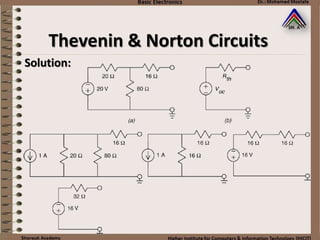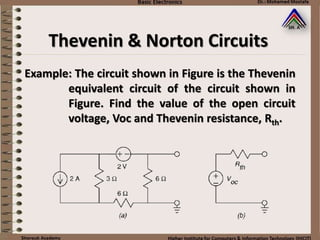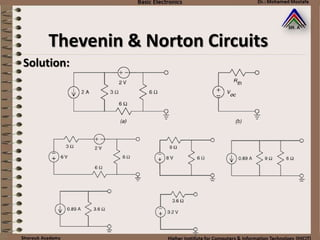1 von 80
Anzeige

### 4 kirchoff law

1. Kirchoff law http://circuits.ece.gatech.edu/
2. Kirchoff law In 1854 Kirchoff announced the two laws that are still the basis for most circuit analysis techniques
3. Kirchoff law Kirchoff Current law (KCL)  Kirchoff’s first Law, known as Kirchoff’s Current Law (KCL), states that current flows uniformly in a circuit (At any node the sum of the currents flowing into the node is exactly equal to the sum of the currents flowing out of the node) I1 + I2 + I3= I4 + I5 = 0  So for the giving node:
4. For the circuit giving, write a KCL equation at every node in the circuit in terms of the current variables whose reference directions are given: Example : I3 I4 I2 I1 IS Kirchoff Current law (KCL) Kirchoff law
5. Node a: I1 + I2 = I3 + Is Node b: I2 = I3 + I4 Node c: Is = I1 + I4 a b c Example : Kirchoff Current law (KCL) Kirchoff law
6. Example : Find current “I” and power “P”? Kirchoff law
7. Kirchoff Voltage law (KVL) V1 = V2 + V3 + V4  Kirchoff’s second Law, known as Kirchoff’s Voltage Law (KVL), states that the sum of the voltages is a closed loop is always equal to zero.  So for the circuit here: Kirchoff law
8. Node Analysis  When analyzing more complex circuits, engineers usually make use of one of the more powerful techniques available.  One of the most popular of these is the “Node Analysis Method”.  The “Node Analysis Method” is a well organized technique which relies on KCL  Equations are written for every unknown node. The method is best illustrated with an example.
9. Example:  Consider the giving circuit.  voltages at node A = +3V, node C is -10V.  Only node B is unknown  Current at node B, by KCL ∑ Iin = ∑ Iout or I1 = I2 + I3  Using Ohm’s Law, VB = -1 V Node Analysis
10. Q:When is the Node Analysis Method most useful? A:When there are only a few unknown node voltages. Node Analysis
11. VS V1 V3 V4 ++ + + _ _ _ _ Example: The circuit below contains four elements and a voltage source. Write a sufficient set of KCL equations to fully constrain all the currents in the circuit. Let the current flowing into the element with voltage V1 be I1, etc.? I1 = I4 I1 + I2 + iS = 0 KCL at node a: KCL at node b: a b Node Analysis
12. Quiz: Find current I3 in the circuit below, using Node Analysis Method? -1.64 mA +1.64 mA -3.82 mA I1 = (3-VB)/R1 = (3-VB)/1000 I2 = (VB+9)/R2 = (VB+9)/1500 I3 = (VB)/R3 = (VB)/500 I1 = I2 + I3 VB = -.82 V I3 = -1.64 mA Node Analysis
13. Quiz: Find current I3 in the circuit below, using the Node Analysis Method -1.64 mA +1.64 mA 0 mA Node Analysis
14. Example: Write a KVL equations for the giving circuit? -V3 - V2 = 0 -V1 - VS = 0 VS + V2 + V4 = 0 IS I4 I1 I2 I3 Node Analysis
15. Example: Find the values of the currents for the giving circuit? Is = I1 + I2 I3 = I2 + IV = I2 + 0.2V1 = I2 + 0.2I1*10Ω = I2 + 2I1 Apply KCL: Apply KVL: -10I1 + 5I2 - V = 0 +V + 5I3 = 0 I2 = 0 I1 = Is I3 = 2Is V1 I1 I3 Is I2 Iv=0.2V110Ω 5Ω 5Ω V Node Analysis
16. 20Ω 10Ω5Ω 15Ω + _ V1 + _ V6 __ ++ _ + V3 V2 V4 IS VS I6 Example: In the giving circuit? A. How many nodes are present in the circuit? B. Write a KCL and a KVL equations? V3 = V4 + V2 V1 + V2 = VS V4 = V1 + V6 KVL equations : KCL equations : + = IS V3V2 155 = V2V1 520 V4 10 + - - IS = 0 V4V3 1015 - = I6 + IS V1 20 a b c d a: b: c: d: Node Analysis
17. 4Ω 2Ω 3Ω + _ V0 I2 i4I0 VS IS1Ω I1 i3 VS + 2I1 + I2 = 0 I2 + 3I3 = 4I4 -4I4 - V0 = 0 KVL equations : KCL equations : a b c d a: I0 - I1 = 0 b: I1 - I2 + I3 = 0 c: -I3 - I4 + IS = 0 d: -I0 + I2 + I4 - IS = 0 Example: In the giving circuit? A. How many nodes are present in the circuit? B. Write a KCL and a KVL equations? Node Analysis
18. 2Ω V1 V2 + _VS IS1Ω 2Ω 1Ω + _ V4V3 + _+ _ Example: For the giving circuit write a sufficient set of KCL equations in terms of the voltage variables that are labelled? a b c Node B: 0.5V1 - V2 - V3 = 0 Node C: V2 =0.5V4 + IS Node Analysis
19. Example: In the giving circuit, the voltage source is a DC source with VS = 60V and the current source supplies a constant current of 3mA. (a) Compute the voltage V2 and V4. (b) Determine the amount of power supplied by each source. (c) Determine the amount of power absorbed by each of the four resistors. (d) Verify that the total power supplied by the two sources is equal to the total power absorbed by the four resistors. (V2- 60)/10 + V2 /15+ (V2 – V4)/18 = 0 (V4 - V2)/18 = V4 /12 + 3 KCL equations: V2 = V4 = 36 V Ptotal = Vs*(60V-36V)/(10kΩ*103) + IS*10-3 *36V = 252 W Node Analysis
20. Mesh Analysis
21. Mesh Analysis  The most popular technique used to solve complex circuit problems is the “Mesh Method”  A “mesh” is one of the smallest circuit loops. For example, in the typical circuit shown, there are 3 possible loops – including the outer loop which includes both batteries. But there are only 2 possible meshes and
22. Mesh Analysis The Mesh Method is a well organized technique which relies on KVL. Mesh (or loop) equations are written for every mesh in terms of the unknown currents. Again, the method is best illustrated by a example.
23. Example: Consider the circuit, with 2 mesh and Find I1, I2 and I3. Assume i1, i2 are CW and i1 > i2. let units be V, mA and kΩ Note : Voltage polarities are assigned to all resistors, based on the assumption made for the mesh current and Ohm’s Law Mesh Analysis
24.  A good tip is to start in the lower-left hand corner and proceed clockwise around the mesh (loop). The first sign encounter determine the sign for that term. And, by KVL, the sum of all terms for each mesh equals zero.  The mesh equations are straight-forward except for the (i1-i2)R3 term. Both I1 and I2 flow thru R3, but we assumed that i1 > i2, thus the current is i1-i2. Note, if any of our assumptions were wrong, we will just get negative answers. For mesh (1): For mesh (2): -3 + i1R1 + (i1-i2)R3 = 0 -(i1-i2)R3 + i2R2 - 10 = 0 Mesh Analysis
25. -3 + i1R1 + (i1-i2)R3 = 0 -3 + i1R1 + (i1-i2)R3 = 0 3i1 - i2 = 3 -(i1-i2)R3 + i2R2 - 10 = 0 -(i1-i2)(1) + 3i2 - 10 = 0 -i1 + 4i2 = 10 i2 = 3 mA i1 = 2 mA I2 = i2 = 3 mA I1 = i1 = 2 mA i3 = I1 – i2 = -1 mA Mesh Analysis
26. Q: What happens if a current source is in one mesh? A: The current source defines the current for that mesh (I1 = 5A). Mesh Analysis
27. Example: Find i1, i2. Assume i1, i2 are CW and i1 > i2. let units be V, mA and kΩ KVL for Mesh (1) -9 + i1(1) + (i1-1)3 = 0 i1 + 3i1 = 9+3 4i1 = 12 i1 = 3 mA KVL for Mesh (2) i2 = 1 mA Mesh Analysis
28. Quiz : Find current I3 in the circuit below, using the Mesh Analysis Method. -1.63 mA +1.63 mA -3.26mA Mesh Analysis
29. i2 = 5.45 mA Answer: I3 = i1 – i2 = -1.63 mA Mesh Analysis i1 = 3.82 mA 1) -3V + i1R1 + (i1 – i2) R3 = 0 -3 + (1)i1 + 0.5(i1 – i2) = 0 1,5i1 – 0.5i2 = 3 2) - (i1 – i2) R3 + i2 R2 – 9V = 0 - (i1 – i2)(0.5) + 1.5i2 – 9 = 0 - 0.5 i1 + 2i2 = 9
30. Example: Solve for the current through the 5 ohm resistor and the current through the 18V source using Mesh-Current Analysis. Mesh Analysis
31. Solution: KVL for i1: KVL for i2: -18V + 5(i1-i2) + 4(i1-i3) + 1(i1) = 0 10i1- 5i2-4i3 - 18V = 0 -12 + 2i2 -5(i1 – i2) + 3(i2 - i3) = 0 KVL for i3: -4(i1 –i3) – 3(i2 –i3) +2i3 = 0 i1 = 7.02A , i2 = 6.28A , i3 = 5.21A The current through the 5 ohm resistor is (i1 - i2) = 0.74A Mesh Analysis
32. Solution: KCL for V1: (V1-V4)/2 + (V1-V2)/3 + V1/2 = 0 Example: Solve for the current through the 5 ohm resistor and the current through the 18V source using Node-Voltage Analysis. KCL for V2: (V2-V3)/5 + V2/4 + (V2-V1)/3 = 0 KCL for V3: (V4-V1)/2 + (V3-V2)/5 + (V5)/1 = 0 V4 - V3 = 12V V3 - V5 = 18V Now we have 5 equations and 5 unknowns Mesh Analysis
33. Example: Solve for the current through the 5 ohm resistor and the current through the 4V source using Mesh-Current Analysis. KVL loop 2: KVL loop 1: i1= 5A 5(i2-i1)+10(i2-i4)+2(i2-i3) = 0 KVL loop 3: -5i1 + 17i2 – 2i3 = 0 i3 = -2A KVL loop 4: 10i4 + 4V + 10(I4-I2) = 0 -10i2 + 20i4 = -4 Now, we have 4 equations with 4 unknown ++ -- Mesh Analysis
34. Example: Solve for the current through the 5 ohm resistor and the current through the 4V source using Node-Voltage Analysis. KCL at V1: V1 = 17.08V V2 = 7.17V -5A + V1/5 + (V1-V2)/10 + [V1-(V2+4)]/10 = 0 KCL at V2: (V2-V1)/10 + V2/2 - 2A + [V2-(V1-4)]/10 = 0 Mesh Analysis
35. Example: Solve for the current through the 5 ohm resistor and the voltage over the 3A source using Mesh- Current Analysis. Mesh Analysis
36. KCL at V1: V1 = 48.625V V2 = 33 V V3 = 48.75V  The current through the 5 ohm resistor can be found by Ohm's law: I = (V1 - V2)/5 = 3.125A  The voltage over the 3A source is simply V1, or 48.625V -3A + (V1-V2)/5 + (V1-V3)/1 = 0 KCL at V2: (V2-V1)/5 + V2/3 + (V2-V3)/2 =0 KCL at V3: (V3-V2)/2 + (V3-V1)/1 - 8A = 0 Solution: Mesh Analysis
37. Supermesh Analysis Determine the values of the mesh currents, i1 and i2 for this circuit: (i1-i2) = 1.5  i1 = i2 + 1.5 9i1+V -12= 0 (1) 3i2 +6i2 -V= 0 (2) Combining equations (1) and (2) gives: 9i1+ 9i2 = 12 9i1+3i2+6i2-12 = 0 9i1 + 9i2 = 12 (i1-i2) = 1.5  i1 = i2 + 1.5 i1 = 1.4167 A , i2 = -83.3 mA Method #1 Method #2
38. Mesh 1: Mesh 2: Mesh 3: -VS+R1 × (I1-I2) + R3 × (I1-I3)=0 R1 × (I2-I1) + R2 × I2 - VIS=0 R3 × (I3-I1) + VIS +R4 × I3 =0 Mesh 1: -VS+R1 × (I1-I2) + R3 × (I1-I3)=0 supermesh : R3 × (I3-I1) + R1 × (I2-I1) + R2 × I2 + R4 × I3 =0 2I3-3I1 + 8I2 =0 Example: Solve the circuit using mesh analysis method and find the power of sources: Assume that VS=10V , IS=4A , R1=2Ω , R2=6Ω , R3=1Ω , R4=1Ω. Supermesh Analysis
39. Superposition The basic concept of the “Superposition Method” is to analyze a circuit, one source at a time Using the superposition method, we remove all the (independent) sources, except one, and analyze the circuit for that one.
40. Then we repeat the procedure for another source, and so on. Finally, the net result is founds by summing all the single-source results. Again, the technique is best explained with an example The Superposition Method us useful for analyzing circuits with multiple power sources. Superposition
41.  Before moving on, we need to understand the idea of “removing” power source. Consider he typical circuit shown – with two voltage sources:  If we were to remove one source, and still have a complete circuit, we replace the voltage source with a short circuit. Superposition
42.  Before moving on, we need to understand the idea of “removing” power source. Consider he typical circuit shown – with two voltage sources:  Similarly, if we have a circuit with two current sources and we remove one source, but still have complete circuit, we replace the current source with an open circuit Superposition
43. Reinforcing this point: to remove a voltage a voltage source replace with a short. To remove a current source replace it with an open Superposition
44. Example : find the voltage at node B. Superposition
45. We remove the 10V source and find node voltage B for only the 3V source. Answer: Superposition Using the Node Method : I1 = I2 + I3 3 – VB VB VB 2 3 1 = + 3(3 – VB) 2VB 6VB 6 6 6 = + 9 = 11 VB VB = 9/11 V
46. Next, we remove the 3V source and find node voltage B for only the 10V source. Answer: Using the Node Method : I1 = I2 + I3 0 – VB VB – (-10) VB 2 3 1 = + –3VB 2(VB +10) 6VB 6 6 6 = + 20 = -11 VB VB = -20/11 V Superposition
47. The net result is the sum of these two calculations: VBNET = -1 V Which are the same results we obtained using the Node and Mesh Methods. i2 = 3 mA i1 = 2 mA i3 = -1 mA and Answer: Superposition
48. Q: Does the Superposition Method only work for computing voltages? A: The Superposition Method work for computing currents? Superposition
49. Example: Find I3 using Superposition Superposition I3 due to 12v source only: I3 due to 4mA source only: I3(12v) = 12V / (1 + 2) = 12V / 3 = 4mA I3(4mA) = (4)(1||2) / (2) = (4)(0.67)/2 = -1.33mA I3(NET) = 4 – 1.33 = 2.67 mA
50. Quiz: Find current Io in the circuit below, using the Superposition Method. -0.84 mA +0.84 mA 0 mA Superposition
51. Answer: 0.84mA Superposition Let units be: V, mA & KΩ For 3V source only, with 5V source removed: RT1 = (1)(1.5)/(1 + 1.5) = 0.6 KΩ IT = 3V / 2.6KΩ = 1.15 mA Using the CDR, I0(3v) = 1.15(0.6)/1 = 0.69ma ↓
52. Superposition Answer: 0.84mA For 5V source only, with 3V source removed: RT2 = (1)(2)/(1 + 2) = 0.66 KΩ IT = 5V / 2.16KΩ = 2.31 mA Io(5v) = 2.31V(0.66)/1 = 1.53 mA ↑ So Io(net) = Io(3v) + Io(5v) = 0.69 ↓ - 1.53 ↑ Io = -8.84 ↑
53. Thevenin & Norton Circuits The basic premise behind Thevenin and Norton circuit analysis is that any complex circuit can be replaced by a simple Thevenin Equivalent or Norton Equivalent
54. A Thevenin Equivalent circuit consists of a single voltage source and a single series resistor Warning:  it is important to identify the point of view for the equivalent circuit.  Usually it is identified by saying “at port A-B” Thevenin & Norton Circuits
55. The Thevenin Equivalent voltage is simply the open-circuit (no load) voltage at the port of interest. The load resistor, if any, must be removed Thevenin & Norton Circuits Using VDR: VTH = VOC = 1V VOC = (3V)(1Ω)/(1Ω + 2Ω) = 1V
56. A Norton Equivalent circuit consists of a single current source and a single parallel resistor Warning:  it is important to identify the point of view for the equivalent circuit.  Usually it is identified by saying “at port A-B” Thevenin & Norton Circuits
57. The Norton Equivalent current is simply the short-circuit current at the port of interest. The load resistor, if any, must be removed Thevenin & Norton Circuits ISC = 3V/2Ω = 1.5A IN = ISC = 1.5A
58. The Thevenin equivalent resistor equals the Norton equivalent resistor and may be determined in two ways: First, RTH = RN = VOpen-Circuit / Ishort-Circuit Thevenin & Norton Circuits RTh = VOC/ISC = 1V/1.5A RTh= 0.67Ω
59. Second, the Thevinin = Norton equivalent resistance is the resistance at the port of interest with the load resistor removed and with all sources removed Thevenin & Norton Circuits RTh = 1Ω || 2 Ω = (1)(2)/(1+2) RTh= 0.67Ω
60. The Thevinin Equivalent circuit may then be constructed: it consists of the Thevenin voltage in a series with the Thevenin Resistor Thevenin & Norton Circuits VOC= VTh =1V ISC= IN =1.5A RTh = RN = 0.67Ω
61. The Norton Equivalent circuit may also be constructed: it consists of the Norton current in parallel with the Norton Resistor. Thevenin & Norton Circuits VOC= VTh =1V ISC= IN =1.5A RTh = RN = 0.67Ω
62. Example: In the shown circuit treat resistor R3, as the load resistor and find the open-circuit voltage VBG. Also find the short-circuit current IBG. Alternatively, find RBG with both sources removed and with R3 removed: RBG = 2kΩ // 3 kΩ = 1.2 kΩ I = 13V / 5KΩ = 2.6 mA I SC = 3V / 2KΩ - 10V/3KΩ = 1.5 = 3.33 = -1.83mA VOC = 3V – (2.6)(2) = -2.2 V RTH = VOC / ISC = -2.2 V / -1.83 mA = 1.2 KΩ Thevenin & Norton Circuits
63. The Thevenin Equivalent circuit can be drawn. Reconnect R3 to terminal B-G and calculate current I3 = -1mA Thevenin & Norton Circuits Thevenin Equivalent VTH = VOC = -2.2V RTH = 1.20KΩ I3 = -2.2V / (1.2 + 1.0KΩ) = -1.0 mA
64.  Likewise, The Norton Equivalent circuit can be drawn. R3 is then reconnected to the terminal B-G and I3 is determined using the CDR : I3 = -1mA  The Thevenin and Norton Equivalent circuit produce the same answer for I3 as the other methods. Thevenin & Norton Circuits ISC = -183mA RN = RTH = 1.20KΩ RT = (1.2)(1)/(1 + 1.20) = 1.20/2.20 = .55KΩ I3 = -(1.83)(.55KΩ)/1KΩ = -1.0 mA Norton Equivalent
65. Thevenin & Norton Circuits  There is another reason for finding the Thevenin or Norton Equivalent of a complex circuit  IT can be shown (but we will not) that the maximum power a circuit can deliver to a load resistor is when the load resistor equals the source resistor.  Or, the maximum power output occurs when the load resistor equals the Thevenin resistance. RL = RTH for Maximum Output Power For this example, the maximum power that can be delivered to RL is when RL = RTH = 1.2KΩ or ROUT MAX = I2 RL = (0.92)2 x 1.2 = 1.0 mW
66. Q: IS it easy to convert a Thevenin Equivalent circuit to a Norton Equivalent circuit? A: Yes, just use Ohm’s Law. Thevenin & Norton Circuits RTh = RN IN= VTh /RTh
67. Thevenin & Norton Circuits I = (V1 – V2)/(5 + R1) = (9-1.5)/(5+10) = 7.5/15 = - 0.5mA VTH = V2+ IR1 = 1.5+(0.5)(10) = 1.5+5 = 6.5V RTH = R2 + (5R1)/(5 + R1) = 2.5+(5)(10)/(5 + 10) = 2.5+3.33 RTH = 5.83KΩ
68. Quiz: Convert the Thevenin circuit below to a Norton equivalent circuit. How much is the maximum power the circuit can supply to load resistor RL IN = 9A, RN = 9Ω & Pmax = 27W IN = 6A, RN = 6Ω & Pmax = 54W IN = 4A, RN = 3Ω & Pmax = 12W Thevenin & Norton Circuits
69. Answer: Thevenin & Norton Circuits INort = 4A, RNort = 3Ω & Pmax = 12W Thevenin Norton IN = ISC = 12V/3Ω = 4A RN = RTH = 3Ω RL = RTH = 3Ω Pmax @ RL = I2RL = = (2)2(3) = 12W IL = 12V/6Ω = 2A
70. Example: for the circuit in figure. It is given that R1=20K, R2=1K, and A1=50. Solve for the Thevenin and Norton equivalent circuits seen looking into the output terminals Thevenin & Norton Circuits
71. Thevenin & Norton Circuits Thevenin equivalent Circuit VDR + Req superposition VOC = VS R2/(R1+R2) + A1i1(R1||R2) i1 = VS/(R1+R2) - A1i1R2/(R1+R2) i1 = VS/(R1+ (1 + A1)R2) VOC = VS R2/(R1+R2) + A1 (R1||R2)(VS/(R1+ (1 + A1)R2)) = 0.718VS
72. Thevenin & Norton Circuits Norton equivalent Circuit iSC = VS/R1 + A1i1 KCL i1 = VS/R1 R2 excluded iSC = VS/R1 + A1(Vs/R1) = VS(1+ A1)/R1 = VS(1+ 50)/1KΩ iSC = 2.55 x 10-3 Vs Rout = VOC/iSC = 0.718Vs/(2.55x10-3VS) = 282Ω
73. Example: Find the Thévenin equivalent with respect to the 7k ohm resistor. Thevenin & Norton Circuits
74. Solution:  Remove the 7KΩ, since it is not part of the circuit we wish to simplify.  Find VTH, the voltage across the terminals (voltage across the 3k ohm).  1k and 2k are parallel. 1k || 2k = (1k*2k) / (1k+2k) = 2M/3k = 2/3k = 667Ω  Now use VDR to compute VTH across the 3k ohm. VTH = [3k/(667+3k)] * 5V = 4.1V  Find the Thevenin Resistance by remove all sources and computing the total resistance across the terminals.  The voltage sources is shorted. Rth = 1k||2k||3k = 545 ohms  The final Thevenin equivalent is then: Thevenin & Norton Circuits
75. Example: Find the Norton Equivalent with respect to the 3K resistor in the middle of the circuit, i.e., the 3K resistor itself should not be part of the equivalent that you compute. Thevenin & Norton Circuits
76. Solution: • To find the Norton with respect to the 3K, we remove the 3K out of the circuit • The circuit to the left of the 3K is already a Norton equivalent, where the Norton current is -10 mA (because it is facing down). The resistance is infinite. That is, when you open the current source to deactivate it, the 1K and 2K are left disconnected. • The circuit to the right of the 3K is already a Thevenin, where the voltage is 6V and the equivalent resistance is 9K. Converting to a Norton, we get Norton current of 0.667 mA and a resistance of 9K. • Now combine the two Nortons. The total current will be: -10mA + 0.667mA = -9.33 mA. • The total resistance is infinite in parallel with 9K, which is simply 9K. Thevenin & Norton Circuits
77. Example: The circuit shown right is the Thevenin equivalent circuit of the circuit shown left. Find the value of the open circuit voltage, Voc and Thevenin resistance, Rth. Thevenin & Norton Circuits
78. Solution: Thevenin & Norton Circuits
79. Example: The circuit shown in Figure is the Thevenin equivalent circuit of the circuit shown in Figure. Find the value of the open circuit voltage, Voc and Thevenin resistance, Rth. Thevenin & Norton Circuits
80. Solution: Thevenin & Norton Circuits
Anzeige